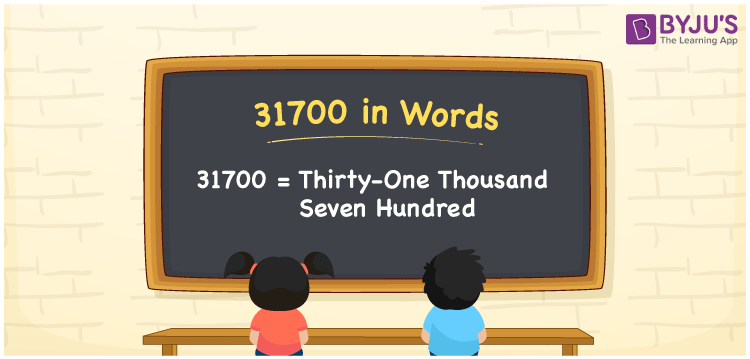# 31700 in Words

31700 in words can be written as Thirty-one Thousand Seven Hundred. The fundamental concepts in Mathematics like counting or count can be learnt efficiently here. If you buy gold worth Rs. 31700, then you can say that “I bought gold ornaments for Thirty-one Thousand Seven Hundred Rupees”. To write numbers in words, the English alphabet is used. The numbers in words concept is explained here in a simple way to improve the conceptual knowledge of students. The 31700 can be read as “Thirty-one Thousand Seven Hundred” in English.

 31700 in words Thirty-one Thousand Seven Hundred Thirty-one Thousand Seven Hundred in Numbers 31700

## 31700 in English Words## How to Write 31700 in Words?

Students can learn about the expanded form and the place value chart of 31700. Five digits are present in the number 31700. With the help of the place value chart given below, students will be able to understand the concepts with ease.

 Ten Thousands Thousands Hundreds Tens Ones 3 1 7 0 0

31700 can be written in expanded form as:

3 x Ten Thousand + 1 x Thousand + 7 × Hundred + 0 × Ten + 0 × One

= 3 x 10000 + 1 x 1000 + 7 × 100 + 0 × 10 + 0 × 1

= 30000 + 1000 + 700

= 31700

= Thirty-one Thousand Seven Hundred

Hence, 31700 in words is written as Thirty-one Thousand Seven Hundred.

31700 is a natural number that precedes 31701 and succeeds 31699.

31700 in words – Thirty-one Thousand Seven Hundred

Is 31700 an odd number? – No

Is 31700 an even number? – Yes

Is 31700 a perfect square number? – No

Is 31700 a perfect cube number? – No

Is 31700 a prime number? – No

Is 31700 a composite number? – Yes

## Frequently Asked Questions on 31700 in Words

Q1

### How to write 31700 in words?

31700 can be written in words as “Thirty-one Thousand Seven Hundred”.
Q2

### How to write Thirty-one Thousand Seven Hundred in numbers?

Thirty-one Thousand Seven Hundred in numbers can be written as 31700.
Q3

### Is 31700 an odd or even number?

31700 is an even number as it is completely divisible by 2.